Test: Matrix

# Test: Matrix

Test Description

## 15 Questions MCQ Test Topic-wise Tests & Solved Examples for IIT JAM Mathematics | Test: Matrix

Test: Matrix for Mathematics 2023 is part of Topic-wise Tests & Solved Examples for IIT JAM Mathematics preparation. The Test: Matrix questions and answers have been prepared according to the Mathematics exam syllabus.The Test: Matrix MCQs are made for Mathematics 2023 Exam. Find important definitions, questions, notes, meanings, examples, exercises, MCQs and online tests for Test: Matrix below.
Solutions of Test: Matrix questions in English are available as part of our Topic-wise Tests & Solved Examples for IIT JAM Mathematics for Mathematics & Test: Matrix solutions in Hindi for Topic-wise Tests & Solved Examples for IIT JAM Mathematics course. Download more important topics, notes, lectures and mock test series for Mathematics Exam by signing up for free. Attempt Test: Matrix | 15 questions in 60 minutes | Mock test for Mathematics preparation | Free important questions MCQ to study Topic-wise Tests & Solved Examples for IIT JAM Mathematics for Mathematics Exam | Download free PDF with solutions
 1 Crore+ students have signed up on EduRev. Have you?
Test: Matrix - Question 1

### Let,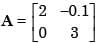and A–1 =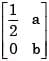Then (a + b) =

Detailed Solution for Test: Matrix - Question 1

We know AA-1 = I2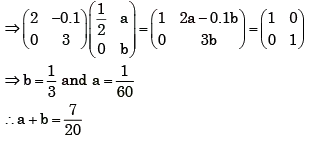Test: Matrix - Question 2

### Given an orthogonal matrix A =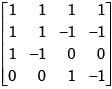[AAT]-1 is

Detailed Solution for Test: Matrix - Question 2

We know
AAt = I4
[AAT]-1 = [I4]-1 = I4

Test: Matrix - Question 3

### The rank of the matrix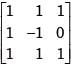is

Detailed Solution for Test: Matrix - Question 3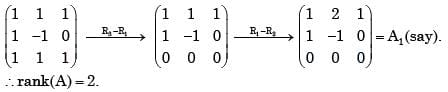Test: Matrix - Question 4

The eigen values of a skew-symmetric matrix are

Detailed Solution for Test: Matrix - Question 4

Solution :- Let  A  be real skew symmetric and suppose  λ∈C  is an eigenvalue, with (complex) eigenvector  v . Then, denoting by  H  hermitian transposition,

λvHv=vH(λv)=vH(Av)=vH(−AHv)=−(vHAH)v=−(Av)Hv=−(λv)Hv=−λ¯vHv

Since  vHv≠0 , as  v≠0 , we get

λ=−λ¯

so  λ  is purely imaginary (which includes 0). Note that the proof works the same for a antihermitian (complex) matrix.

With a completely similar technique you can prove that the eigenvalues of a Hermitian matrix (which includes real symmetric) are real.

Test: Matrix - Question 5

Rank of the matrix given below is: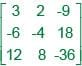Detailed Solution for Test: Matrix - Question 5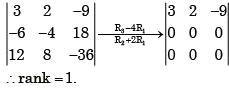Test: Matrix - Question 6

The rank of a 3 x 3 matrix C (=AB), found by multiplying a non-zero column matrix A of size 3 x 1 and a non-zero row matrix B of size 1 x 3, is

Detailed Solution for Test: Matrix - Question 6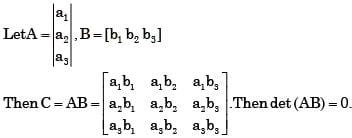Then also every minor or order 2 is also zero.
∴ rank (C) = 1

Test: Matrix - Question 7

A is a 3 x 4 real matrix and A x = b is an inconsistent system of equations. The highest possible rank of A is

Detailed Solution for Test: Matrix - Question 7

Highest possible rank of A= 2 ,as   Ax = b is an inconsistent system.

Test: Matrix - Question 8

Match the items in columns I and II.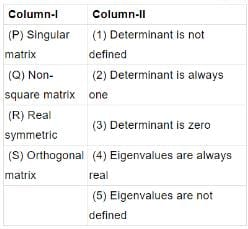Detailed Solution for Test: Matrix - Question 8

(P) Singular matrix → Determinant is zero
(Q) Non-square matrix → Determinant is not defined
(R) Real symmetric → Eigen values are always real
(S) Ortho gonal → Det erminant is always one

Test: Matrix - Question 9

[A] is a square matrix which is neither symmetric nor skew-symmetric and [A]T is its transpose. The sum and difference of these matrices are defined as [S] = [A] + [A]T and [D] = [A] -[A]T, respectively. Which of the following statements is True?

Test: Matrix - Question 10

Given matrix [A] =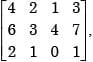the rank of the matrix is

Detailed Solution for Test: Matrix - Question 10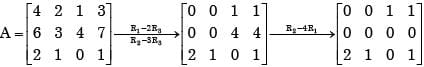∴ Rank (A) = 2

Test: Matrix - Question 11

Real matrices [A]3 x 1, [B]3 x 3, [C]3 x 5,[D]5 x 3, [E]5 x 5 and [F]5 x 1  are given. Matrices [B] and [E] are symmetric.
Following statements are made with respect to these matrices.
1. Matrix product [F]T [C]T [B] [C] [F] is a scalar.
2. Matrix product [D]T [F] [D] is always symmetric.
With reference to above statements, which of the following applies?

Detailed Solution for Test: Matrix - Question 11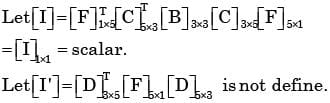Test: Matrix - Question 12

Consider the matrices X (4 × 3), Y (4 × 3) and P (2 × 3). The order or P (XTY)–1PT] T will be

Detailed Solution for Test: Matrix - Question 12

When two matrices (x,y) and (a,b) are to be multiplied, the multiplication is possible

when y = a, and the resultant matrix is of the order (x * a)

XT = The matrix in which rows and columns are interchanged

{(2 x 3) x [(3x4) x (4x3)]-1 x (3x2)}= { (2x3) x (3x3) x (3x2) }T = { (2x3) x (3x2) }T = 2 x 2

Test: Matrix - Question 13

The inverse of the 2  x 2 matrix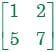is,

Detailed Solution for Test: Matrix - Question 13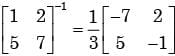Test: Matrix - Question 14

The product of matrices (PQ)-1 P is

Detailed Solution for Test: Matrix - Question 14

(PQ)-1P = Q-1P-1P = Q-1

Test: Matrix - Question 15

A square matrix B is skew-symmetric if

Detailed Solution for Test: Matrix - Question 15

BT = – B

## Topic-wise Tests & Solved Examples for IIT JAM Mathematics

27 docs|150 tests(Scan QR code)